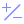#Round (PQL)

Returns the value of a number rounded to the nearest integer.

• Returned Output: Numeric
• Library: PQL \ Common \ Math
• Version: 2018.00.000
• Compatibility:
• Can be combined with and other PQL function throughout the application.
• It CANNOT be used with MDX or VBA functions. But it can be used on MDX-based content in other parts of the application.

#### Syntax

Round( <Numeric> , )

* Click on the function's arguments above for more details on the input values.

• The numeric value cane be any type of number: integer, float etc.
• The position is a positive integer reflecting the number of decimals to keep in the result. Zero is assumed if not supplied.
##### Different Function types
• This function is like the Excel round function.
• Also see Ceiling and Floor functions.

#### Examples

This example returns "1"

Round(0.95,0)

This example returns "-5.15"

Round(-5.153,2)